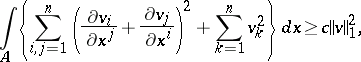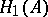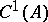# Korn inequality

Jump to: navigation, search

An inequality for vector functions,, and their derivatives, defined in some bounded domainof:(1)

where(2)

The Korn inequality is also valid for vector functions in the spaceobtained by completing the spacewith respect to the norm (2). The inequality (1) is sometimes called the second Korn inequality; the first Korn inequality being inequality (1) without the second term on the left.

The inequality was proposed by A. Korn (1908) in order to obtain an a priori estimate for the solution of non-homogeneous equations of elasticity theory.